# 基于多步态零速检测的MEMS IMU室内定位技术Indoor Positioning Technology Based on MEMS IMU Using Multi-Step Zero Speed Detection

• 全文下载: PDF(3310KB)    PP.28-38   DOI: 10.12677/JSTA.2019.72004
• 下载量: 393  浏览量: 1,307

An indoor positioning method based on low cost MEMS inertial sensor is studied. Aiming at the shortcomings of traditional single threshold zero speed interval detection algorithm, a new algo-rithm suitable for multi gait is proposed. The low cost inertial measurement unit (IMU) is fixed on the foot. Firstly, according to the output of the acceleration, motion state of the pedestrian can be determined. Then, under the threshold condition of the corresponding state, the zero speed interval detection is carried out by combining the three methods of specific force, specific force variance and angular velocity. At the same time, the Kalman filter is designed to estimate the system attitude error and the speed position error in the static time and the system is compensated by the feedback correction. Finally, the low cost MEMS IMU is used f to verify the experiment. The results show that the positioning accuracy can be maintained at about 3%.

1. 引言

MEMS IMU以其自主性强、输出频率高、短时精度高、低成本、小体积等优点成为室内导航的理想设备。基于MEMS IMU的室内导航系统中最常用的结构是Foxlin提出的鞋绑式结构  ；文献  将惯性传感器放在腰上，其数据平稳动态性弱，但是行走过程中零速区间不明显，对积累误差的修正较为复杂。零速修正(Zero Velocity Update, ZUPT)和零角速度修正(Zero Angular Rate Update, ZARU)是惯导系统中常用的修正算法，其中最为关键的就是零速区间的检测。

2. MEMS惯性传感器室内定位系统原理

MEMS惯性传感器室内定位系统主要由三部分组成，分别为导航算法部分，零速检测部分以及误差修正部分。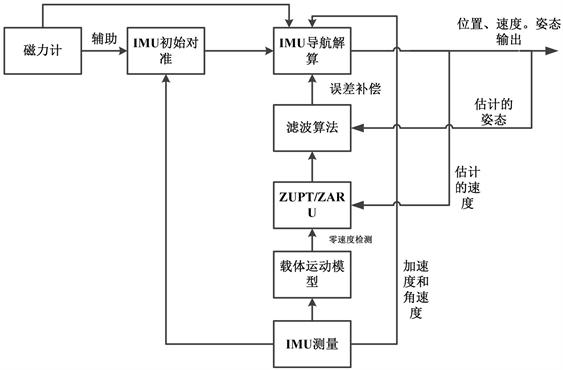Figure 1. MEMS inertial sensors positioning

3. 适应于多步态零速区间检测算法

3.1. 判断阈值选取Table 1. Selection of threshold parameters

$t{h}_{a\mathrm{min}}$$t{h}_{a\mathrm{max}}$ 为比力模值的阈值， $t{h}_{\sigma }$ 为比力方差的阈值， $t{h}_{w}$ 为加速度模值的阈值。

3.2. 判断当前运动状态

${f}_{i}|=\sqrt{{f}_{xi}^{2}+{f}_{yi}^{2}+{f}_{zi}^{2}}$ (1)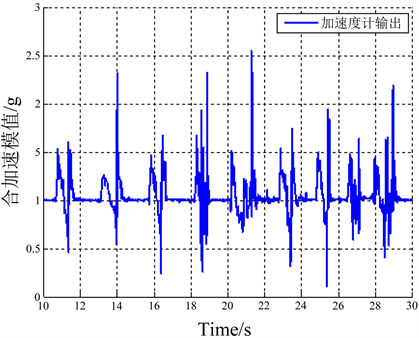Figure 2. Combined acceleration of normal state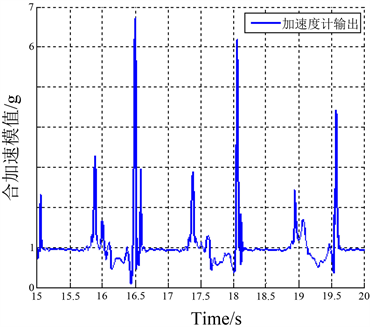Figure 3. Combined acceleration of climbing stairs

${f}_{\mathrm{max}}-{f}_{\mathrm{min}}<3\text{\hspace{0.17em}}\text{g}$ 是正常行走的范围；

$3\text{\hspace{0.17em}}\text{g}<{f}_{\mathrm{max}}-{f}_{\mathrm{min}}<4\text{\hspace{0.17em}}\text{g}$ 是快速行走的范围；

$4\text{\hspace{0.17em}}\text{g}<{f}_{\mathrm{max}}-{f}_{\mathrm{min}}<7\text{\hspace{0.17em}}\text{g}$ 是上下楼梯的范围；

${f}_{\mathrm{max}}-{f}_{\mathrm{min}}>7\text{\hspace{0.17em}}\text{g}$ 是大踏步或跳跃的范围。

3.3. 零速区间检测

$\text{condition}1=\left\{\begin{array}{cc}\begin{array}{l}1\\ 0\end{array}& \begin{array}{l}t{h}_{a\mathrm{min}}<|{f}_{k}| (2)

${\sigma }_{{f}_{k}}^{2}=\frac{1}{2m+1}{\underset{j=k-m}{\overset{k+m}{\sum }}\left({f}_{j}-{\stackrel{¯}{f}}_{k}\right)}^{2}$ (3)

$\text{condition}2=\left\{\begin{array}{cc}\begin{array}{l}1\\ 0\end{array}& \begin{array}{l}{\sigma }_{{f}_{k}} (4)

$\text{condition}3=\left\{\begin{array}{cc}\begin{array}{l}1\\ 0\end{array}& \begin{array}{l}|{w}_{k}| (5)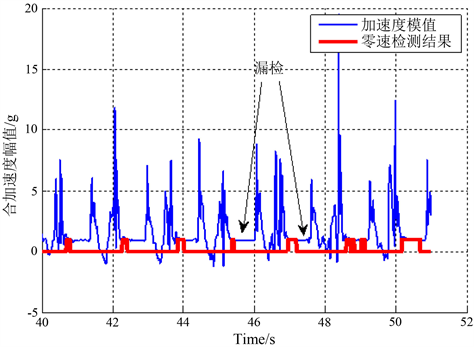Figure 4. Detection result in big strides by a single threshold method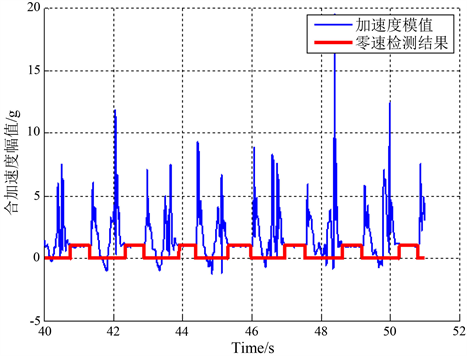Figure 5. Detection result in big strides by the method suitable for multi gait

4. 零速修正滤波算法

$X={\left[\begin{array}{cc}\begin{array}{cc}\delta \phi & \begin{array}{ccc}{\epsilon }^{b}& \delta {p}^{n}& \delta {v}^{n}\end{array}\end{array}& {\nabla }^{b}\end{array}\right]}^{T}$ (6)

${\epsilon }^{b}$ 表示三个轴向的陀螺漂移；

$\delta p$ 表示导航坐标系下导航解算的位置误差；

$\delta {v}^{n}$ 表示导航坐标系下导航解算的速度误差；

${\nabla }^{b}$ 表示三个轴向的加速度误差。

${Z}_{k}={\left[\delta {v}^{n}\right]}^{T}={\left[{v}^{n}\right]}^{T}-{\left[\begin{array}{ccc}0& 0& 0\end{array}\right]}^{T}$ (7)

$\left\{\begin{array}{l}\stackrel{˙}{X}\left(t\right)=F\left(t\right)X\left(t\right)+W\left(t\right)\\ {Z}_{k}={H}_{k}{X}_{k}+{V}_{k}\end{array}$ (8)

${H}_{k}=\left[\begin{array}{ccccc}{0}_{3×3}& {I}_{3×3}& {0}_{3×3}& {0}_{3×3}& {0}_{3×3}\\ {0}_{3×3}& {0}_{3×3}& {0}_{3×3}& {I}_{3×3}& {0}_{3×3}\end{array}\right]$ (9)

5. 定位实验验证

5.1. 硬件介绍Table 2. Hardware performance index

N表示该项没有具体指标。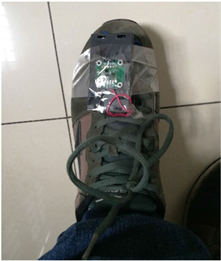Figure 6. MPU9250 physical drawing

5.2. 平面行走实验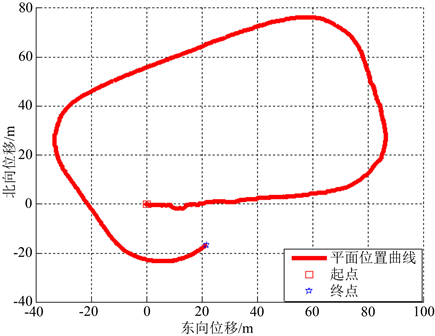Figure 7. Position curve of plane walking corrected by single threshold and ZUPT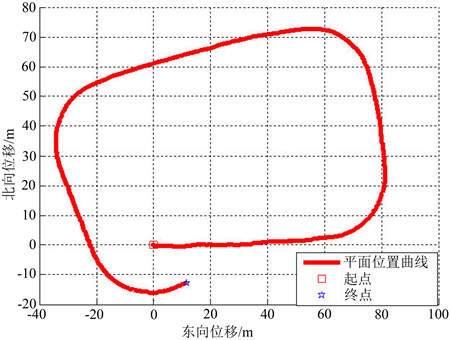Figure 8. Position curve of plane walking corrected by the multi gait algorithmTable 3. Contradistinction of plane walking result in two ways

5.3. 三维上下楼实验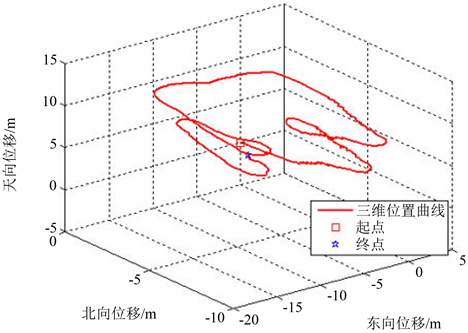Figure 9. Position curve of stair walking corrected by single threshold and ZUPT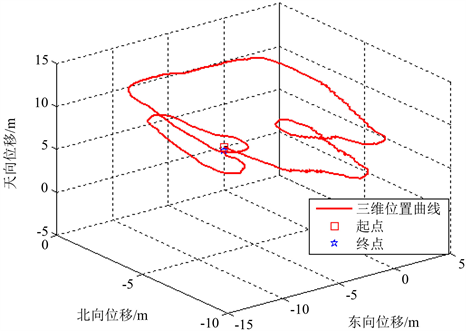Figure 10. Position curve of stair walking corrected by the multi gait algorithmTable 4. Contradistinction of stair walking result in two ways

6. 结论

  Jo, K., Chu, K. and Sunwoo, M. (2012) Interacting Multiple Model Filter-Based Sensor Fusion of GPS with In-Vehicle Sensors for Real-Time Vehicle Positioning. IEEE Transactions on Intelligent Transportation Systems, 13, 329-343.  刘成. LBS定位技术研究与发展现状[J]. 导航定位学报, 2013(1): 78-83.  Foxlin, E. (2005) Pedestrian Tracking with Shoe-Mounted Inertial Sensors. IEEE Computer Graphics and Applications, 25, 38-46.  Alvarez, J.C., López, A.M., González, R.C., et al. (2012) Pedestrian Dead Reckoning with Waist-Worn Inertial Sensors. Proceedings of Instrumentation and Measurement Technology Conference (12MTC), Graz, Austria, 13-16 May 2012, 24-27.  Fourati, H. (2015) Heterogeneous Data Fusion Algorithm for Pedestrian Navigation via Foot-Mounted Inertial Measurement Unit and Complementary Filter. IEEE Transactions on Instrumentation Measurement, 64, 221-229. https://doi.org/10.1109/TIM.2014.2335912  张金亮, 秦永元, 梅春波. 基于MEMS惯性技术的鞋式个人导航系统[J]. 中国惯性技术学报, 2011, 19(3): 253-256.  王立兵, 杨松普, 罗巍, 等. 足部安装MEMS-IMU个人导航系统[J]. 中国惯性技术学报, 2016, 24(4): 460-463.  王普, 潘凯, 任明荣, 等. 基于伪自适应阈值零速检测法的室内个人导航系统[J]. 北京工业大学学报, 2015(9): 1308-1313.  Bebek, O., Suster, M.A., Rajgopal, S., et al. (2010) Personal Navigation via Shoe Mounted Inertial Measurement Units. Proceedings of International Conference on Intelligent Robots and Systems (IROS), Taipei, 18-22 October 2010.  Ramón, A., Ruiz, J., et al. (2012) Accurate Pedestrian Indoor Navigation by Tightly Coupling Foot-Mounted IMU and RFID Measurements. Instrumentation and Measurement, 11, 178-189.  Skog, I., Handel, P., Nilsson, J., et al. (2010) Zero-Velocity Detection—An Algorithm Evaluation. IEEE Transactions on Biomedical Engineering, 57, 2657-2666.  Zhang, H., Yuan, W., Shen, Q., et al. (2015) A Handheld Inertial Pedestrian Navigation System with Accurate Step Modes and Device Poses Recognition. IEEE Sensors Journal, 15, 1421-1429. https://doi.org/10.1109/JSEN.2014.2363157